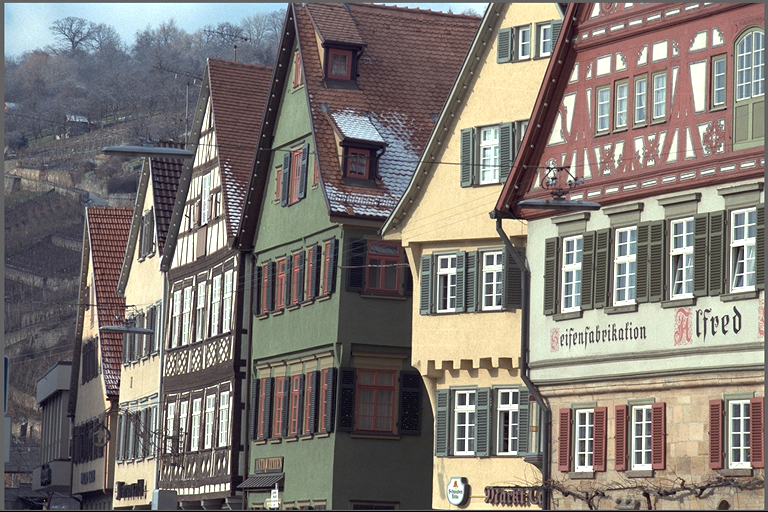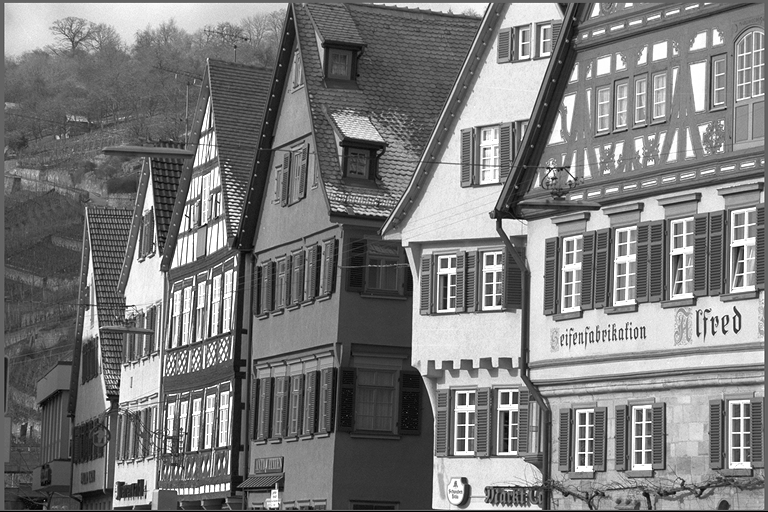## VPI - Vision Programming Interface

#### 2.2 Release

Convert Image Format

# Overview

The Convert Image Format is used to convert an image with a given format into another format. It handles both color spec, format and depth conversions. The algorithm also supports input range conversion, when one is required to map, for instance, an unsigned char $$[0,255]$$ image into signed short $$[-32768,32767]$$ range.

Color Input Grayscale Output# C API functions

For list of limitations, constraints and backends that implements the algorithm, consult reference documentation of the following functions:

Function Description
vpiSubmitConvertImageFormat Converts the image contents to the desired format, with optional scaling and offset.

# Implementation

The algorithm is implemented as a pixel-wise conversion function that reads in the input pixels, applies a conversion-dependent series of transformations and writes the result to the output image in the same position. User inputs are:

• input image created with requested input type
• output image created with requested output type
• flags specify how type casts will be performed, see clamp/cast
• scale and offset to be used in range conversions, see range.

Several types of conversion are available:

• grayscale $$\leftrightarrow$$ color
• grayscale $$\leftrightarrow$$ grayscale (useful in depth and range conversions)
• color $$\leftrightarrow$$ color (e.g. YUV to RGB and vice-versa)

The color formats available are:

• YUV color spec:
• RGB color spec:
• without alpha channel:
• with alpha channel:

The single channel, grayscale formats available are:

• luma formats:
• non-color integral formats:
• non-color floating point format:

Non-color formats are to be used when the information stored isn't represented as a color, such as temperature, speed, etc. For conversions, they are considered to be grayscale with extended range.

The following table shows which combinations of input and output image types are available for conversion.

in
out

# Conversion Formulas

The following sections describe how input value is converted into output. In general, these conversions amount to color spec, depth, channel order (swizzle), adding or removing alpha channel, down- or up-sampling transformations. These are represented as conversion pipelines made out of basic processing blocks defined below.

## Channel Depth Conversion

Channel depth conversion is represented by the block aptly named "depth" and is defined by the following sub-pipeline:

 depth $$=$$ range $$\rightarrow$$ round $$\rightarrow$$ clamp/cast
• range: input is converted to floating point (fp32) and the following formula is applied:

$f(x) = \text{scale} \times x + \text{offset}$

If scale==1 and offset==0, a shortcut is taken and no operation (not even conversion to floating point) is performed.

• round: round to the nearest integer, with halfway cases being rounded away from zero, e.g round(0.5) == 1.0 and round(-0.5) == -1.0.
• clamp/cast: operation controlled by the passed flags:
• VPI_CONVERSION_CAST : cast input to output type like regular C cast or C++'s static_cast would do. Underflows and overflows will behave as described by C specification (including undefined behavior). This is used when it's known that input range fits into output and maximum performance is needed.
• VPI_CONVERSION_CLAMP : the value is clamped so that overflows and overflows will map to output type's maximum and minimum values, respectively. The result is then cast to the output type. When output type is floating point, clamp behaves like cast.

When applied to multiple channels such as RGB, the operation is performed on each channel independently.

## Channel Order Conversion

This is represented by the following block:

 swizzle

It's used to permute (or swizzle) input type's channel order. Used in conversions from/to color specs that can be represented in multiple ways, like RGB and BGR. The color spec conversion functions assume a pre-determined channel order. In order to use them, channels must be reordered.

## Conversion Between YUV and RGB

For RGB $$\leftrightarrow$$ YUV conversions, VPI uses the ITU-R BT.601 625-line specification. It's the same standard used by JPEG File Interchange Format (JFIF).

To precisely establish the conversion, let's define the following constants:

\begin{align} K_r &= 0.299 \\ K_g &= 0.587 \\ K_b &= 0.114 \\ K_{c_b} &= 1.772 \\ K_{c_r} &= 1.402 \\ \end{align}

For notation convenience, we're assuming that $$U$$ and $$V$$ correspond to $$C_b$$ and $$C_r$$ respectively. This assumption doesn't hold in general.

The conversion blocks can be defined as:

 rgb2yuv

\begin{align} Y(r,g,b) &= \text{round}(r K_r + g K_g + b K_b)\big|^{255}_{0} \\ C_b(r,g,b) &= \text{round}((-r K_r - g K_g + b (1 - K_b )) / K_ {c_b} + 128)\big|^{255}_{0} \\ C_r(r,g,b) &= \text{round}((r (1-K_r) - g K_g - b K_b) / K_{c_r} + 128)\big|^{255}_{0} \end{align}

These functions expect $$r,g,b \in [0,255]$$

 yuv2rgb

\begin{align} R(y,c_b,c_r) &= \text{round}(y+K_{c_r}(c_r-128))\big|^{255}_{0} \\ G(y,c_b,c_r) &= \text{round}(y-[K_b K_{c_b} (c_b-128) + K_r K_{c_r} (c_r-128)] / K_g)\big|^{255}_{0} \\ B(y,c_b,c_r) &= \text{round}(y + K_{c_b} (c_b - 128))\big|^{255}_{0} \end{align}

These functions expect $$y,c_b,c_r \in [0,255]$$

The notation $$X\big|^{N} _ {M}$$ represents clamping X's underflows and overflows to M and N respectively.

The round function follows the definition here.

## Conversion Between RGB and Grayscale

Conversion from RGB to grayscale follows the same specification used for conversion from RGB to YUV, but just returning the luma component. Hence, using the same constants defined here.

 rgb2gray

$Y(r,g,b) = \text{round}(K_r \times r + K_g \times g + K_b \times b)\big|^{255}_{0}$

For grayscale to RGB the conversion is simply:

 gray2rgb

$f(x) = (x,x,x)$

## Up-/Down-Sampling

For image formats that includes subsampled planes like VPI_IMAGE_FORMAT_NV12, the following block definitions are needed:

 2x downsample

$D[x,y] = S[2x,2y]$

 2x upsample

$D[x,y] = S[\lfloor x/2 \rfloor, \lfloor y/2 \rfloor]$

Note
VPI is effectively upsamping using nearest-neighbor sampling. In a future version it'll use bilinear upsampling.

## Alpha Channel Handling

Depending on input and output pixel type, i.e. whether it's required to remove or add an alpha channel, the following block might be used:

 alpha
• add alpha: append an opaque alpha channel to input pixel, e.g. RGB becomes RGBA. For integral channel types, the new alpha channel's value is the maximum representable of its type, e.g. 255 for 8-bit unsigned integer. Currently VPI doesn't support alpha channel on other channel types.
• remove alpha: simply discards the alpha channel, e.g. RGBA becomes RGB.
• do nothing: when input and output don't have alpha channel.

# Conversion Pipelines

This section defines how input pixel is converted to output. It uses the basic conversion blocks defined in previous section.

## Grayscale from/to Grayscale

 input $$\rightarrow$$ depth $$\rightarrow$$ output

## Grayscale to NV12/NV24

 input $$\rightarrow$$ depth $$\rightarrow$$ Y plane $$\searrow$$ (128,128) $$\rightarrow$$ UV plane $$\nearrow$$
 output
Note
Since NV12/NV24's pixel depth is 8-bit unsigned, $$(u,v) = (128,128)$$ corresponds to zero saturation.

## NV12/NV24 to Grayscale

 input $$\rightarrow$$ Y plane $$\rightarrow$$ depth $$\rightarrow$$ output

## Grayscale to RGB Space

 input $$\rightarrow$$ depth $$\rightarrow$$ gray2rgb $$\rightarrow$$ swizzle $$\rightarrow$$ alpha $$\rightarrow$$ output

## RGB Space to Grayscale

 input $$\rightarrow$$ swizzle $$\rightarrow$$ alpha $$\rightarrow$$ rgb2gray $$\rightarrow$$ depth $$\rightarrow$$ output

## RGB Space to NV12

 input $$\rightarrow$$ swizzle $$\rightarrow$$ alpha $$\rightarrow$$ depth $$\rightarrow$$ rgb2yuv
 $$\nearrow$$ Y plane $$\searrow$$ $$\searrow$$ UV plane $$\rightarrow$$ 2x downsample $$\nearrow$$
 output

## RGB Space to NV24

 input $$\rightarrow$$ swizzle $$\rightarrow$$ alpha $$\rightarrow$$ depth $$\rightarrow$$ rgb2yuv
 $$\nearrow$$ Y plane $$\searrow$$ $$\searrow$$ UV plane $$\nearrow$$
 output

## NV12 to RGB Space

 input
 $$\nearrow$$ Y plane $$\searrow$$ $$\searrow$$ UV plane $$\rightarrow$$ 2x upsample $$\nearrow$$
 yuv2rgb $$\rightarrow$$ depth $$\rightarrow$$ swizzle $$\rightarrow$$ alpha $$\rightarrow$$ output

## NV24 to RGB Space

 input
 $$\nearrow$$ Y plane $$\searrow$$ $$\searrow$$ UV plane $$\nearrow$$
 yuv2rgb $$\rightarrow$$ depth $$\rightarrow$$ swizzle $$\rightarrow$$ alpha $$\rightarrow$$ output

# Usage

Language:
1. Import VPI module
import vpi
2. Convert the VPI image to S16 format (signed 16-bit), mapping the range from $$[0,255]$$ to $$[-32768,32767]$$.
with vpi.Backend.CPU:
output = input.convert(vpi.Format.S16, scale=257, offset=-32768)
1. Initialization phase
1. Include the header that defines the image format converter function.
Declares functions that handle image format conversion.
2. Create the stream where the algorithm will be submitted for execution.
VPIStream stream;
vpiStreamCreate(0, &stream);
struct VPIStreamImpl * VPIStream
A handle to a stream.
Definition: Types.h:250
VPIStatus vpiStreamCreate(uint64_t flags, VPIStream *stream)
Create a stream instance.
3. Define the input image. Here as an example we're creating a color image with dimensions $$w \times h$$ and NV12 image type.
VPIImage input;
#define VPI_IMAGE_FORMAT_NV12_ER
YUV420sp 8-bit pitch-linear format with full range.
Definition: ImageFormat.h:206
struct VPIImageImpl * VPIImage
A handle to an image.
Definition: Types.h:256
VPIStatus vpiImageCreate(int32_t width, int32_t height, VPIImageFormat fmt, uint64_t flags, VPIImage *img)
Create an empty image instance with the specified flags.
4. Create the output image with the destination image type. In this case, we want to convert the input to 16-bit signed integer grayscale.
int w, h;
vpiImageGetSize(input, &w, &h);
VPIImage output;
#define VPI_IMAGE_FORMAT_S16
Single plane with one 16-bit signed integer channel.
Definition: ImageFormat.h:118
VPIStatus vpiImageGetSize(VPIImage img, int32_t *width, int32_t *height)
Get the image dimensions in pixels.
2. Processing phase
1. Define the conversion parameters to map the range from $$[0,255]$$ to $$[-32768,32767]$$.
cvtParams.scale = 257;
cvtParams.offset = -32768;
VPIConversionPolicy policy
Conversion policy to be used.
float scale
Scaling factor.
VPIStatus vpiInitConvertImageFormatParams(VPIConvertImageFormatParams *params)
Initialize VPIConvertImageFormatParams with default values.
@ VPI_CONVERSION_CLAMP
Clamps input to output's type range.
Definition: Types.h:297
Parameters for customizing image format conversion.
2. Submit the algorithm to the stream, input, output images and other parameters.
vpiSubmitConvertImageFormat(stream, VPI_BACKEND_CPU, input, output, &cvtParams);
VPIStatus vpiSubmitConvertImageFormat(VPIStream stream, uint64_t backend, VPIImage input, VPIImage output, const VPIConvertImageFormatParams *params)
Converts the image contents to the desired format, with optional scaling and offset.
@ VPI_BACKEND_CPU
CPU backend.
Definition: Types.h:92
3. Optionally, wait until the processing is done.
vpiStreamSync(stream);
VPIStatus vpiStreamSync(VPIStream stream)
Blocks the calling thread until all submitted commands in this stream queue are done (queue is empty)...
3. Cleanup phase
1. Free resources held by the stream and the input and output images.
vpiImageDestroy(output);
void vpiImageDestroy(VPIImage img)
Destroy an image instance.
void vpiStreamDestroy(VPIStream stream)
Destroy a stream instance and deallocate all HW resources.

For more information, see Convert Image Format in the "C API Reference" section of VPI - Vision Programming Interface.

# Performance

For information on how to use the performance table below, see Algorithm Performance Tables.
Before comparing measurements, consult Comparing Algorithm Elapsed Times.
For further information on how performance was benchmarked, see Performance Benchmark.

Note
Although VIC supports clamp conversion policy only, the table below shows performance numbers even for cast policy. Internally it's still using clamp. On VIC, it wouldn't make a difference even if it supported cast.
-